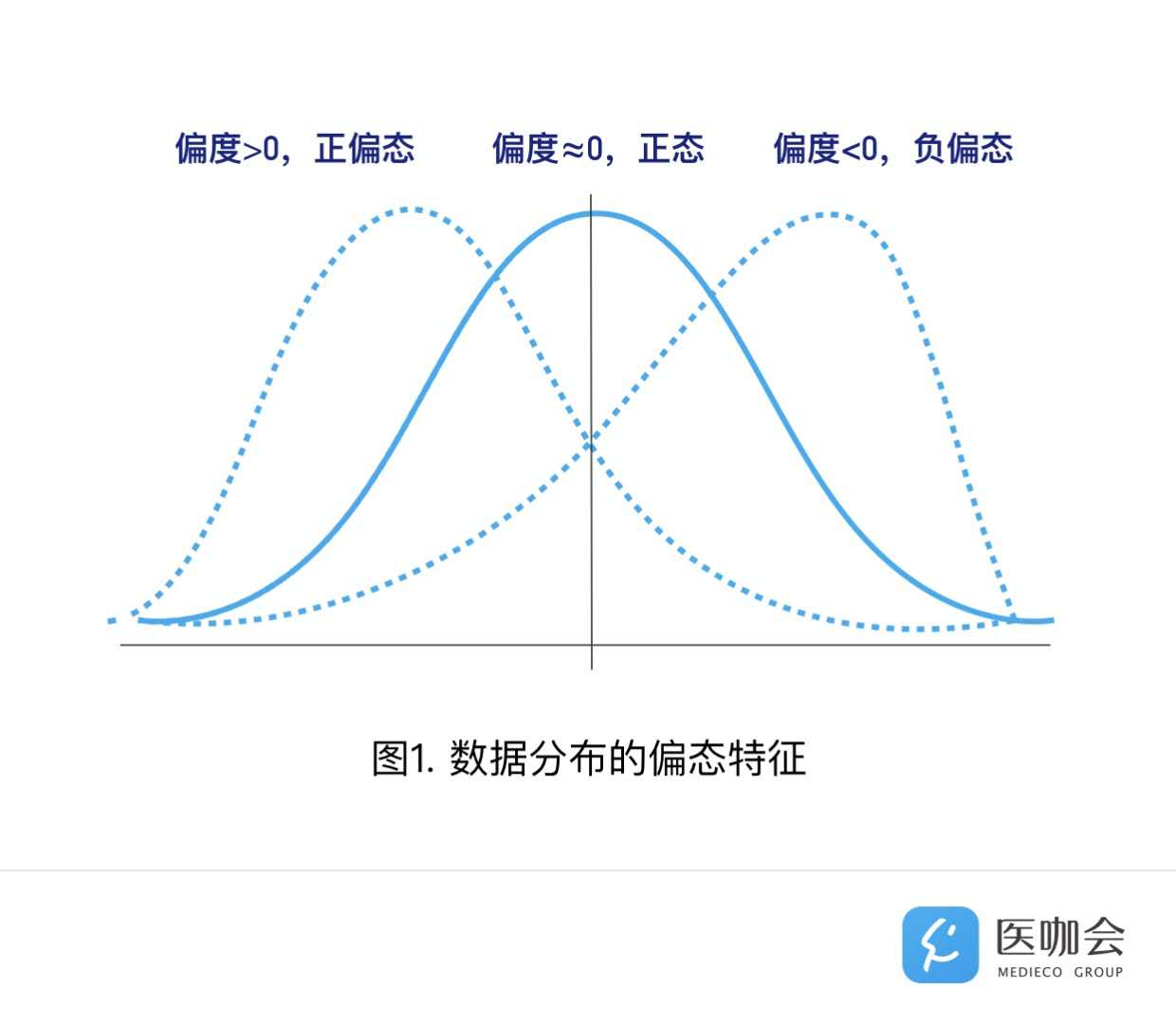# 正态分布的转换

• 取根号
• 取对数
• 取倒数

``````# 生成正态分布数据
normal <- rnorm(100,mean = 5, sd = 2.5)
plot(normal)````````````# 从直方图可以看出数据符合正态分布
hist(normal)````````````# 从QQ plot也可以看出来
qqnorm(normal)````````````# 数据的平方根
normal_square <- normal ^ 2

# 现在的直方图
hist(normal_square)````````````# 数据的幂值
normal_exp <- exp(normal)

hist(normal_exp)````````````# 数据的幂值
normal_reciprocal <- 1/normal

hist(normal_reciprocal)````````````# 轻度负偏态
normal_square_rev <- max(normal_square)+1-normal_square
hist(normal_square_rev)````````````# 中度负偏态
normal_exp_rev <- max(normal_exp)+1-normal_exp
hist(normal_exp_rev)````````````# 重度负偏态
normal_reciprocal_rev <- max(normal_reciprocal) + 1 - normal_reciprocal
hist(normal_reciprocal_rev)``````• 不是任何非正态数据都可以进行正态转换；
• 如果比较两组数据时对一组进行了正态转换，另一组也应做对应转换；
• 在进行相关分析或线性回归时，要求变量间存在线性关系，如果因变量与某个自变量之间呈现出曲线趋势，此时转换的变量可以是自变量，也可以是因变量，或者两者均可。如果进行了变量变换，则应当重新绘制散点图，以保证线性趋势在变换后仍然存在。
• 在对线性回归模型进行解释时，如果使用函数转换的方法对变量进行了转换，则解释时应按照转换后的变量给予解释，或者可以根据转换时使用的函数关系，倒推原始自变量对原始因变量的效应大小。